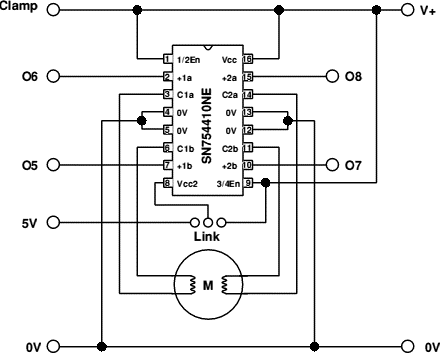SN754410NE double H-bridge and a Raspberry Pipi_bi-polar.zip

 10 REM Pi_bi_step    20 REM Control a bi-polar stepping motor wit the Raspberry Pi    30 REM Jochen Lueg    40 REM http://roevalley.com (bottom of the page)    50 REM Limavady, January 2013    60 REM Version 1.0    70    80 ON ERROR PROCerror    90 PROCinit   100 OFF   110 OSCLI"RMEnsure GPIO 0.00 RMLoad GPIO"   120 OSCLI"RMensure GPIO 0.40 ERROR  Please install the GPIO module"   130 PROCsetupGPIO   140   150   160 PRINT   170 PRINT " Motor controls"   180 PRINT   190 PRINT " Left       . . . . . .  Z"   200 PRINT " Right      . . . . . .  C"   210 PRINT " Stop       . . . . . .  X"   211 PRINT   220 PRINT " Full step  . . . . . .  F"   230 PRINT " Half step  . . . . . .  H"   240 PRINT   250 PRINT " Fastest    . . . . . .  1"   260 PRINT " to"   270 PRINT " Slowest    . . . . . .  9"   280 PRINT   290 PRINT " Press 'Q' to leave the program"   300   310 REPEAT   320   Key\$=INKEY\$(0) : REPEAT UNTIL INKEY(0)=-1   330   IF Key\$="c" OR Key\$="C" Direction\$="Right"   340   IF Key\$="z" OR Key\$="Z" Direction\$="Left"   350   IF Key\$="q" OR Key\$="Q" Direction\$="Finished"   360   IF Key\$="x" OR Key\$="X" Direction\$="Stop"   370   IF Key\$="h" OR Key\$="H" Mode\$="Half"   380   IF Key\$="f" OR Key\$="F" Mode\$="Full"   390   IF Key\$="1" T%=150*S%   :Speed\$="Fast"   400   IF Key\$="2" T%=200*S%   :Speed\$="Fast-1"   410   IF Key\$="3" T%=300*S%   :Speed\$="Fast-2"   420   IF Key\$="4" T%=625*S%   :Speed\$="Fast-3"   430   IF Key\$="5" T%=1250*S%  :Speed\$="Half"   440   IF Key\$="6" T%=2500*S%  :Speed\$="Slow+3"   450   IF Key\$="7" T%=5000*S%  :Speed\$="Slow+2"   460   IF Key\$="8" T%=10000*S% :Speed\$="Slow+1"   470   IF Key\$="9" T%=20000*S% :Speed\$="Slow"   480   IF Direction\$="Right" AND Mode\$="Full" THEN PROCfull_step_right   490   IF Direction\$="Right" AND Mode\$="Half" THEN PROChalf_step_right   500   IF Direction\$="Left" AND Mode\$="Full"  THEN PROCfull_step_left   510   IF Direction\$="Left" AND Mode\$="Half"  THEN PROChalf_step_left   520   IF Direction\$="Stop" PRINTTAB(1,19)"Motor stopped                                 "   530   IF Direction\$="Stop" PROCall_off: Direction\$="Wait"   540   IF Direction\$ <> "Wait" PRINTTAB(1,19);Mode\$;" step. Turning ";Direction\$;" with speed ";Speed\$;"   "   550 UNTIL Direction\$="Finished"   560 PROCclose   570 QUIT   580   590 DEFPROCall_off   600 FOR J%=1 TO 4   610   SYS"GPIO_WriteData",Port%(J%),0   620 NEXT   630 ENDPROC   640   650   660 DEFPROCfull_step_right   670 FOR J%=4 TO 1 STEP-1   680   SYS"GPIO_WriteData",Port%(J%),1   690   SYS"GPIO_WriteData",Port%(J%-1),0: FOR I%=1TO T%:NEXT   700 NEXT   710 ENDPROC   720   730   740 DEFPROCfull_step_left   750 FOR J%=1 TO 4   760   SYS"GPIO_WriteData",Port%(J%),1   770   SYS"GPIO_WriteData",Port%(J%-1),0: FOR I%=1TO T%:NEXT   780 NEXT   800 ENDPROC   810   820   830 DEFPROChalf_step_right   840 FOR J%=4 TO 1 STEP-1   850   SYS"GPIO_WriteData",Port%(J%),1   860   SYS"GPIO_WriteData",Port%(J%-1),0: FOR I%=1TO T%:NEXT   870   SYS"GPIO_WriteData",Port%(J%+1),1: FOR I%=1TO T%:NEXT   880 NEXT   900 ENDPROC   910   920   930 DEFPROChalf_step_left   940 FOR J%=1 TO 4   950   SYS"GPIO_WriteData",Port%(J%),1   960   SYS"GPIO_WriteData",Port%(J%-1),0: FOR I%=1TO T%:NEXT   970   SYS"GPIO_WriteData",Port%(J%+1),1: FOR I%=1TO T%:NEXT   980 NEXT  1010 ENDPROC  1020  1030  1040 DEFPROCinit  1050 W%=0  1060 Direction\$="Stop"  1070 S%=200  1080 T%=S%*150  1090 Speed\$="Fast"  1100 Mode\$="Full"  1140 ENDPROC  1150  1160  1170 DEFPROCerror  1180 PRINT REPORT\$;" at line ";ERL  :  1190 FOR J%=1 TO 4  1200   SYS"GPIO_WriteData",Port%(J%),0  1210 NEXT  1230 END  1240 ENDPROC  1250  1251  1260 DEFPROCsetupGPIO  1270 DIM Port%(5) : Port%()=15,24,23,18,15,24  1280 SYS"GPIO_EnableI2C",0  1290 SYS"GPIO_ExpAsGPIO",2  1300 FOR J%=1 TO 4  1310   SYS"GPIO_WriteMode",Port%(J%),1  1320 NEXT  1340 ENDPROC  1350